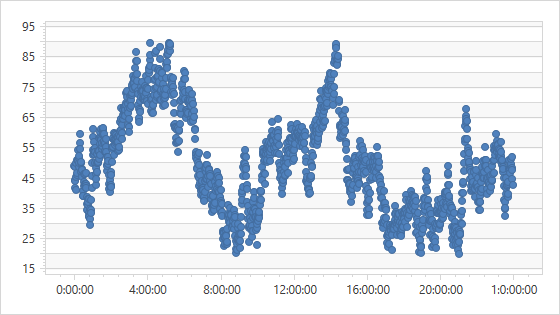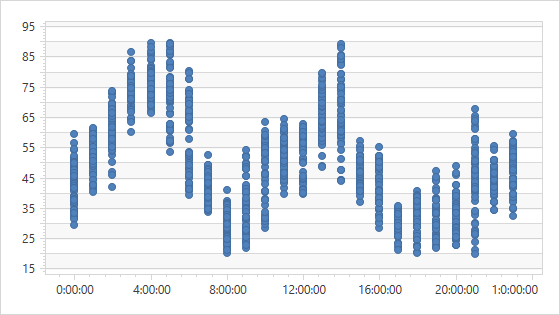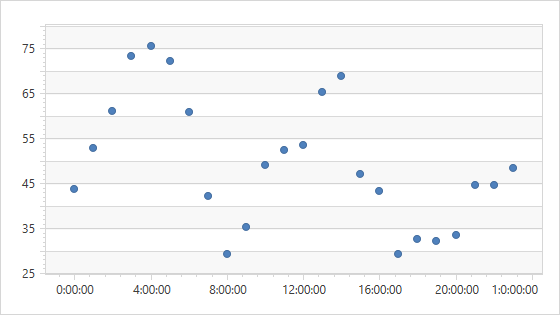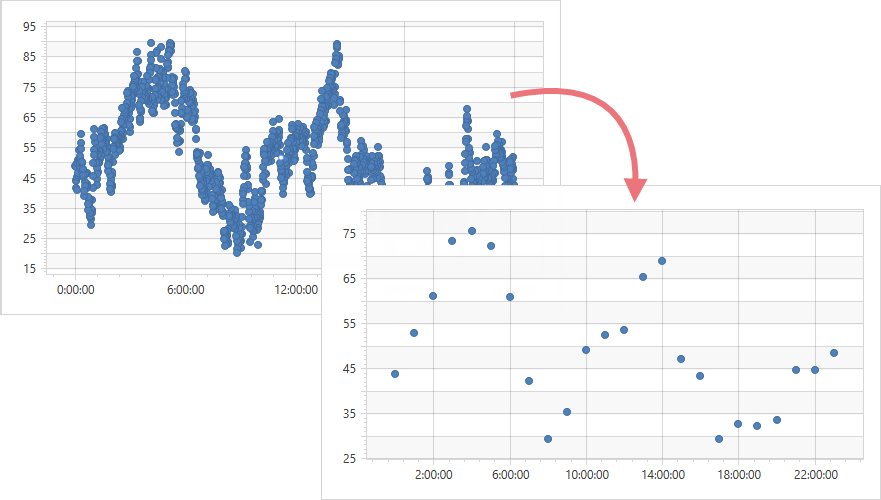21.2 (EAP/Beta)
21.1
20.2
20.1
19.2
19.1
The page you are viewing does not exist in version 19.1. This link will take you to the root page.
18.2
The page you are viewing does not exist in version 18.2. This link will take you to the root page.
18.1
The page you are viewing does not exist in version 18.1. This link will take you to the root page.
17.2
The page you are viewing does not exist in version 17.2. This link will take you to the root page.

# ManualTimeSpanScaleOptions.AggregateFunction Property

Gets or sets the function that is used to aggregate time-span data associated with the axis.

Namespace: DevExpress.Xpf.Charts

Assembly: DevExpress.Xpf.Charts.v21.1.dll

## Declaration

``public AggregateFunction AggregateFunction { get; set; }``

## Property Value

Type Description
AggregateFunction

An aggregate function applied to data.

Available values:

Name Description
None

The aggregate function is not applied.

Average

Aggregates data by calculating the average value for a selected numeric or date-time interval.

Minimum

Aggregates data by calculating the minimum value for a selected numeric or date-time interval.

Maximum

Aggregates data by calculating the maximum value for a selected numeric or date-time interval.

Sum

Aggregates data by calculating the summary for a selected numeric or date-time interval.

Count

Aggregates data by calculating the number of non-null values for a selected numeric or date-time interval.

Histogram

Aggregates data by calculating the number of all points for a selected numeric or date-time interval.

Financial

Aggregates financial data for a selected interval into a single high-low-open-close data point, so that the high value equals the highest value of all data points in this interval; the low value equals the lowest value of all data points; the open value equals the value of the first data point and the close value equals the value of the last data point.

Custom

Aggregates data using the custom CustomAggregateFunction calculator.

## Remarks

The Chart Control can use an aggregate function to group raw data source values and show aggregates as series points. When the AggregateFunction property is not specified, the Chart uses the Average function. For more information, see the Aggregation help topic.

The following images show how changes to the aggregate function and measure unit affect the representation of minute-by-minute chart data.

MeasureUnit = “Minute”; AggregateFunction = “None”:MeasureUnit = “Hour”; AggregateFunction = “None”:MeasureUnit = “Hour”; AggregateFunction = “Average”:## Example

The following example shows how to group minute-by-minute data into data points with a greater measurement unit (“Hour”).``````<Window
xmlns="http://schemas.microsoft.com/winfx/2006/xaml/presentation"
xmlns:x="http://schemas.microsoft.com/winfx/2006/xaml"
xmlns:d="http://schemas.microsoft.com/expression/blend/2008"
xmlns:mc="http://schemas.openxmlformats.org/markup-compatibility/2006"
xmlns:local="clr-namespace:TimeSpanExample"
xmlns:dxc="http://schemas.devexpress.com/winfx/2008/xaml/charts"
x:Class="TimeSpanExample.MainWindow"
mc:Ignorable="d"
Title="MainWindow" Height="450" Width="800">
<Window.DataContext>
<local:ChartViewModel/>
</Window.DataContext>
<Grid>
<dxc:ChartControl>
<dxc:XYDiagram2D>
<dxc:XYDiagram2D.AxisX>
<dxc:AxisX2D>
<dxc:AxisX2D.TimeSpanScaleOptions>
<dxc:ManualTimeSpanScaleOptions GridAlignment="Hour"
GridSpacing="4"
MeasureUnit="Hour"
AggregateFunction="Average"
GridOffset="2"
AutoGrid="False"/>
</dxc:AxisX2D.TimeSpanScaleOptions>
</dxc:AxisX2D>
</dxc:XYDiagram2D.AxisX>
<!-- Other diagram settings. -->
</dxc:XYDiagram2D>
</dxc:ChartControl>
</Grid>
</Window>
``````